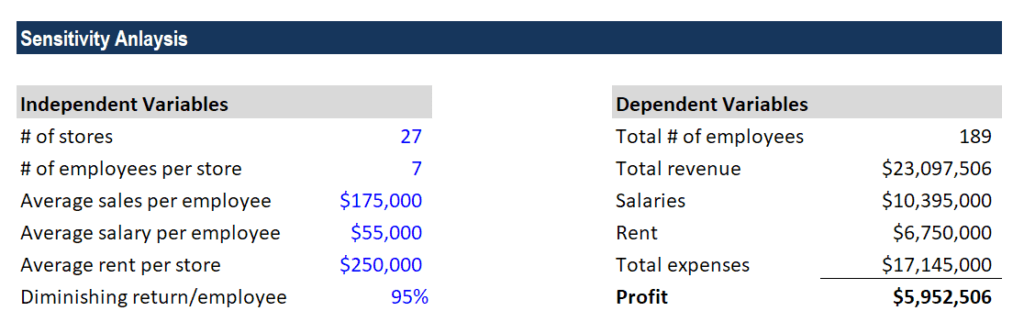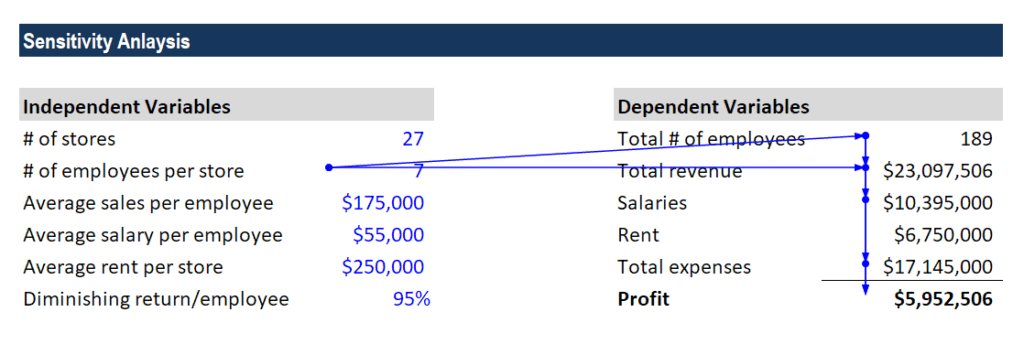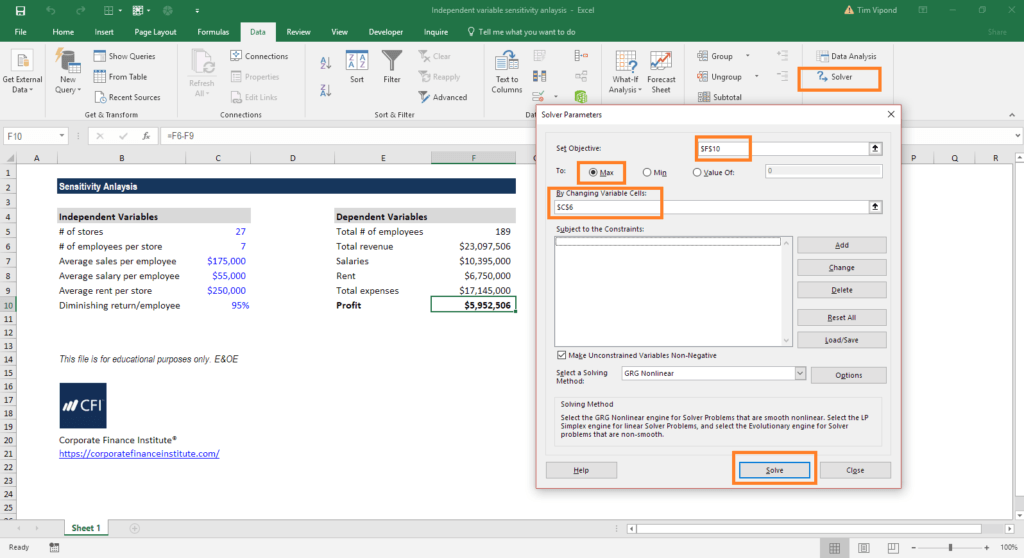# Independent Variable

An input, assumption, or driver that is changed in order to assess its impact on a dependent variable (the outcome)

## What is an Independent Variable?

An independent variable is an input, assumption, or driver that is changed in order to assess its impact on a dependent variable (the outcome). Think of the independent variable as the input and the dependent variable as the output. In financial modeling and analysis, an analyst typically performs sensitivity analysis in Excel, which involves changing assumptions in the model to observe the impact on an output.### Creating an independent variable

When building an Excel model, the analyst needs to think carefully about how to set up an independent variable in the assumptions section. They are the inputs that will drive the model and resulting outputs.

One of the best ways to select assumptions is to perform a root-cause analysis. For example, what causes revenue to fluctuate? Volume and price. What causes the volume to change? The number of products offered and the number of stores. The process continues until the analyst identifies all independent variables that will be used to drive the model.

### Linking independent and dependent variables

Once the variables have been determined and set up in Excel, the next task is to create formulas and functions that determine the outputs. For example, the number of stores times the number of products sold per store times the average price per product equals total revenue.

After the function has been created, the dependent variable, revenue, will be impacted by changes in any of the underlying assumptions and drivers.

At this point, some tests should be performed to ensure the linking is properly set up and the dependent variable changes as expected with the changes in the assumptions.

### Changing the independent variable

Once the model has been built, it’s time to start changing the assumptions and driver to see their impact on the depending variable, and test hypotheses, run scenarios and measure sensitivity.

For example, the analyst may begin to manually change each assumption and record the change in the dependent variable (in this case, revenue).

Another option is to create data tables in Excel that can record the numbers automatically and store them conveniently in a neat table.

Finally, the analyst may run discrete scenarios where a series of changes are made to assumptions with a story tied to them (for example, the company’s “optimistic case” scenario where everything goes well).

### Example of changing an independent variable

Let’s look at an example of how to set up a model that can change an independent variable to test a hypothesis and measure sensitivity to changes.

In this simple model we created, you can see the effect of changing a variable.

The question an analyst would ask is, given all the assumptions, what is the optimal number of employees per store that this company should operate with?

We know that employees generate an average of \$175,000 each, but that there is a diminishing return of 5% to the power of the number of employees per store. Salaries per employee remain the same.Based on the model, the optimal number of employees (the independent variable) is 10 per store, in order to maximize profit (the dependent variable).

### Solver in Excel

The solution can be calculated using Excel’s solver function. Follow the screenshot below to check this solution on your own.

Here are the steps to take:

1. Click on the Data Ribbon.
2. Click on the Solver function.
3. “Set Objective” to the profit cell (F10).
4. Solve for the “Max.”
5. “By changing variable cells” that contain the # of employees (C6).
6. Click Solve.

The result that’s displayed will be 10.

You can also manually test this solution by changing the independent variable until you arrive at the solution above.